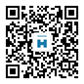021-62677988

# 森活文化2016元旦暨跨年年会在天一山庄盛大召开

2016年注定是不寻常的一年，因为

2016=666+666+666+6+6+6

2016=777+777+77+77+77+77+77+77

2016=888+888+88+88+8+8+8+8+8+8+8+8

2016=999+999+9+9，2016数字的神奇意义，就意味着我们要事事顺心;吃遍天涯海角的美食、生意;一路发发发;最后当然是长长久久，细水长流。新年第一天，要以一个完美的姿态征服这美好的一天。